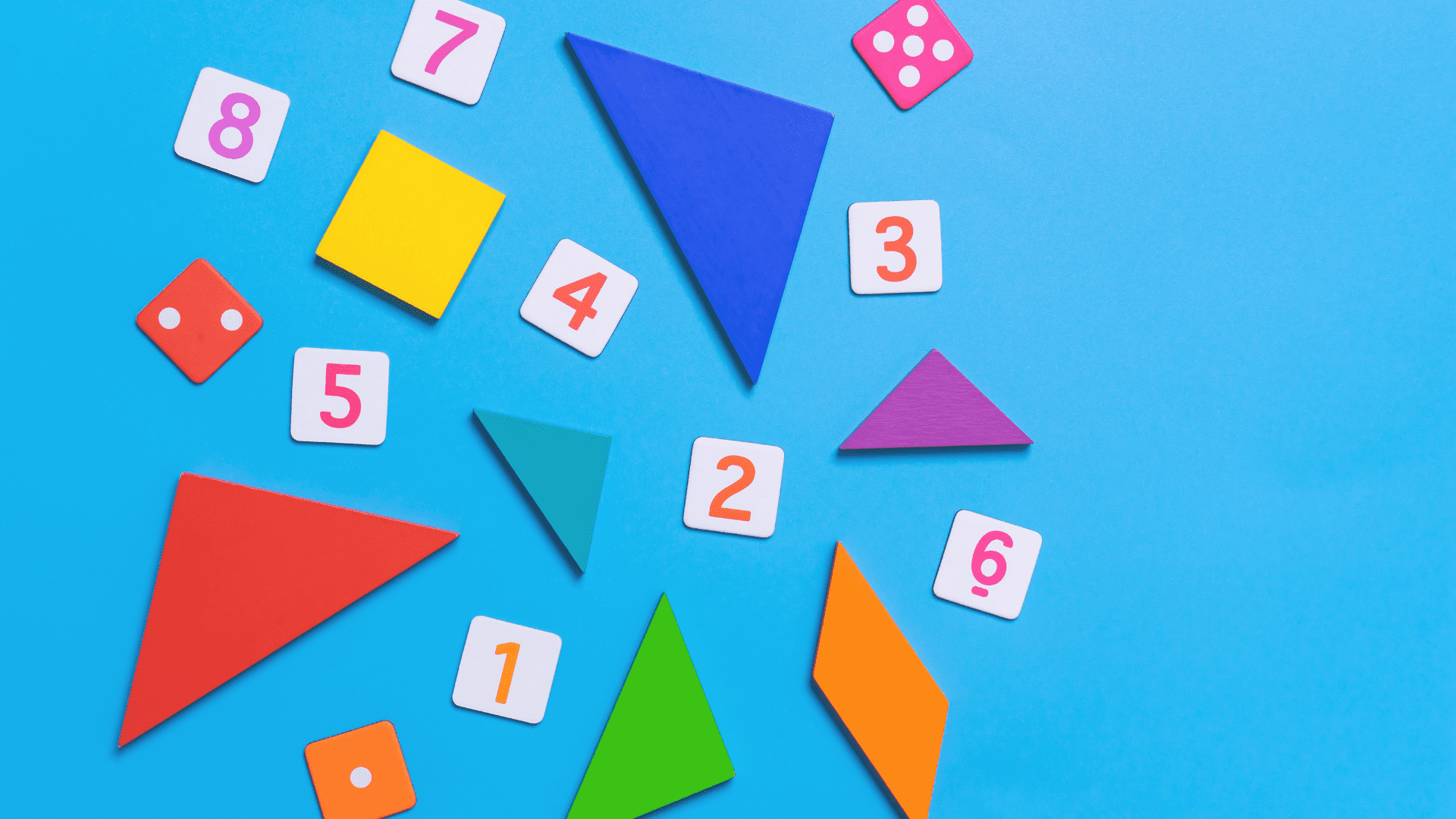# Math is Cool, You’ve Got This!

## Basic Math Operations

From order of operations to basic calculations, we have many free worksheets and games for kids to learn basic math operations: Add, Subtract, Multiply and Divide.

## Fractions

### Browse by Concept: